You are not registered yet. Please click here to register!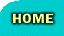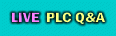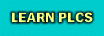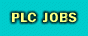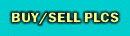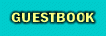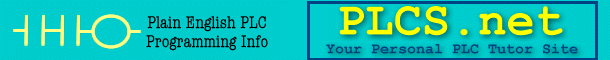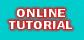This board is for PLC Related Q&A ONLY. Please DON'T use it for advertising, etc.Try our online PLC Simulator- FREE.  Click here now to try it.---------->>>>>Get FREE PLC Programming TipsNew Here? Please read this important info!!!PLCS.net - Interactive Q & AS7 - using Arrays with Multiplex Addressing in ProTool
 User Name Remember Me? Password
 Register FAQ Calendar Downloads PLC Reviews PLCS.net Store Search Today's Posts Mark Forums Read

 Thread Tools Display ModesAugust 11th, 2005, 09:42 AM #1 RMA MemberJoin Date: Sep 2004 Location: North of Hamburg, Germany Posts: 2,052 S7 - using Arrays with Multiplex Addressing in ProTool Since I've suddenly got a couple of weeks spare time due to late delivery of the chargers, I've decided to go through my ProTool project and try to change the addressing of the capacitors in the fault screens from individual addresses in the Multiplex addresses to calls to an array. There are 532 capacitors in the system and since there are two different potential fault states they are each addressed using two different symbols. If I can get this working, I can thus replace 1064 symbols with 42 arrays, which solves my problem with too many variables in one hit. However, after reading the Help files for a few hours, it's becoming obvious to me that I haven't a clue where to begin. What's more, I'm not even sure what questions I need to ask yet, but these are the ones I've thought of so far. 1) Can I do this entirely in ProTool 2) If I manage to create an array in ProTool when I access different points in the array in a Multiplexed address with the form Cap_01_Mod_05, Cap_01_Mod_05, etc. does each access count as another Symbol - if so, we're back at square one! 3) Have I not understood how to use Arrays in ProTool, and it can be done, but not like this - if so, how? 4) As has been suggested before by S7Guy amongst others, one way to do it would be to do the array handling in the PLC and map the result to a single variable in ProTool, rather than using the Multiplex Addressing. If I go this route (and it looks like it might be the easiest, although I'm a little worried about what it might do to the cycle time), how do I populate the arrays? For one situation I'm reading the input signals directly, for the other, I'm reading the results out of a DB. Any pointers to get me moving in the right direction will be most welcome! Cheers Roy Last edited by RMA; August 11th, 2005 at 09:44 AM.August 11th, 2005, 10:12 AM #2 jwall MemberJoin Date: May 2005 Posts: 43 From what I gathered, you could store the information in around 34 DWORDs. You could bring the 34 dwords in, and have 32 bits ( 16 capacitors) for each word. You could then cycle through the 34 words via a mulitplex. I believe in arrays, you can call them out in scripts, using square brackets with an index ..... temp = varray..... Depends on the overhead again. I try and use my PLC as much as possible, and try to bring the packets in implicitly,so that when I access the bits, the are alligned with the item I want. Do you want to send you project over to me? We both seem to be the ones dealing the most with Protool on this forumAugust 11th, 2005, 11:02 AM #3 CharlesM MemberJoin Date: Aug 2005 Location: Arkansas Posts: 1,129 WinCC Flex I do this in WinCC Flex. I read in the DB from the plc then I trigger a script to run on change. I assume you can do the same in Protool 'This Script copys HMI_MUX_Input_1 to internal bool tags 'The plc writes the one variable and the HMI deMux's. 'This reduces the number of Power tags by combining all the bool's into one 16 bit word 'Bit 0 = HMI_Filter_Running 'Bit 1 = HMI_Light_Curtain 'Bit 2 = HMI_Pump_In_Manual 'Bit 3 = Eject_BlowOff_Sol 'Bit 4 = quick_move_equal 'Bit 5 = ram_start_equal 'Bit 6 = table_eject_pos_equal 'Bit 7 = table_fill_pos_equal 'Bit 8 = table_underfill_pos_equal 'Bit 9 = Lift_fill_pos_equal 'Bit 10 = ram_target_pos_equal 'Bit 11 = ram_target_ton_equal 'Bit 12 = ram_slow_dist_equal 'Bit 13 = ram_comp_speed_equal 'Bit 14 = die_float_dist_equal 'Bit 15 = test_time_equal Dim hold, remainder hold = SmartTags("HMI_MUX_Input_1") 'bit 8 remainder = hold Mod 2 hold = hold \ 2 'divide by 2 and remove remainder If remainder = 0 Then SmartTags("table_underfill_pos_equal") = 0 Else SmartTags("table_underfill_pos_equal") = 1 End If 'bit 9 remainder = hold Mod 2 hold = hold \ 2 'divide by 2 and remove remainder If remainder = 0 Then SmartTags("Lift_fill_pos_equal") = 0 Else SmartTags("Lift_fill_pos_equal") = 1 End If 'bit 10 remainder = hold Mod 2 hold = hold \ 2 'divide by 2 and remove remainder If remainder = 0 Then SmartTags("ram_target_pos_equal") = 0 Else SmartTags("ram_target_pos_equal") = 1 End If 'bit 11 remainder = hold Mod 2 hold = hold \ 2 'divide by 2 and remove remainder If remainder = 0 Then SmartTags("ram_target_ton_equal") = 0 Else SmartTags("ram_target_ton_equal") = 1 End If 'bit 12 remainder = hold Mod 2 hold = hold \ 2 'divide by 2 and remove remainder If remainder = 0 Then SmartTags("ram_slow_dist_equal") = 0 Else SmartTags("ram_slow_dist_equal") = 1 End If 'bit 13 remainder = hold Mod 2 hold = hold \ 2 'divide by 2 and remove remainder If remainder = 0 Then SmartTags("ram_comp_speed_equal") = 0 Else SmartTags("ram_comp_speed_equal") = 1 End If 'bit 14 remainder = hold Mod 2 hold = hold \ 2 'divide by 2 and remove remainder If remainder = 0 Then SmartTags("die_float_dist_equal") = 0 Else SmartTags("die_float_dist_equal") = 1 End If 'bit 15 remainder = hold Mod 2 hold = hold \ 2 'divide by 2 and remove remainder If remainder = 0 Then SmartTags("test_time_equal") = 0 Else SmartTags("test_time_equal") = 1 End If 'bit 0 remainder = hold Mod 2 hold = hold \ 2 'divide by 2 and remove remainder If remainder = 0 Then SmartTags("HMI_Filter_Running") = 0 Else SmartTags("HMI_Filter_Running") = 1 End If 'bit 1 remainder = hold Mod 2 hold = hold \ 2 'divide by 2 and remove remainder If remainder = 0 Then SmartTags("HMI_Light_Curtain") = 0 Else SmartTags("HMI_Light_Curtain") = 1 End If 'bit 2 remainder = hold Mod 2 hold = hold \ 2 'divide by 2 and remove remainder If remainder = 0 Then SmartTags("HMI_Pump_In_Manual") = 0 Else SmartTags("HMI_Pump_In_Manual") = 1 End If 'bit 3 remainder = hold Mod 2 hold = hold \ 2 'divide by 2 and remove remainder If remainder = 0 Then SmartTags("Eject_BlowOff_Sol") = 0 Else SmartTags("Eject_BlowOff_Sol") = 1 End If 'bit 4 remainder = hold Mod 2 hold = hold \ 2 'divide by 2 and remove remainder If remainder = 0 Then SmartTags("quick_move_equal") = 0 Else SmartTags("quick_move_equal") = 1 End If 'bit 5 remainder = hold Mod 2 hold = hold \ 2 'divide by 2 and remove remainder If remainder = 0 Then SmartTags("ram_start_equal") = 0 Else SmartTags("ram_start_equal") = 1 End If 'bit 6 remainder = hold Mod 2 hold = hold \ 2 'divide by 2 and remove remainder If remainder = 0 Then SmartTags("table_eject_pos_equal") = 0 Else SmartTags("table_eject_pos_equal") = 1 End If 'bit 7 remainder = hold Mod 2 ''hold = hold \ 2 'divide by 2 and remove remainder If remainder = 0 Then SmartTags("table_fill_pos_equal") = 0 Else SmartTags("table_fill_pos_equal") = 1 End IfAugust 11th, 2005, 11:29 AM #4 RMA MemberJoin Date: Sep 2004 Location: North of Hamburg, Germany Posts: 2,052 @jwall, Sure, I can copy the project over. Need to do it by e-mail though because it's just over 3 MB. Can you PM me your e-mail address? @CharlesM, Ouch, I'm not very fit in Visual Basic. All I know is that it is possible to script in VB in ProTool, but I've never tried it. I guess with WinCC Flexible creeping up on us, if it's anything like normal WinCC where half the programming seems to be done in VB, maybe I better resign myself to learning it!I'll have to have another look at your program tomorrow, it's a bit heavy for me at this time of day!Thanks RoyAugust 12th, 2005, 07:39 AM   #5
JesperMP
Lifetime Supporting Member + ModeratorJoin Date: Feb 2003
Location: Copenhagen.
Posts: 13,624
Quote:
 'This reduces the number of Power tags by combining all the bool's into one 16 bit word
Charles,
Win CC Flex allows you to make arrys of tags. Each array, no matter how big, only counts as one power tag.
Isnt that script of yours redundant ?
__________________
JesperSee my profile interests for Q&ACurrently Active Users Viewing This Thread: 1 (0 members and 1 guests)
 Thread ToolsShow Printable VersionEmail this Page Display ModesLinear ModeSwitch to Hybrid ModeSwitch to Threaded ModePosting Rules You may not post new threads You may not post replies You may not post attachments You may not edit your posts BB code is On Smilies are On [IMG] code is On HTML code is Off
 Forum Jump User Control Panel Private Messages Subscriptions Who's Online Search Forums Forums Home PLCS.net - Interactive Q & A     Announcements     LIVE PLC Questions And Answers     Practice Posting Here     Archives Casual TalkSimilar Topics Thread Thread Starter Forum Replies Last Post RMA LIVE PLC Questions And Answers 9 May 3rd, 2005 10:11 AM RMA LIVE PLC Questions And Answers 0 May 3rd, 2005 06:40 AM RMA LIVE PLC Questions And Answers 45 May 3rd, 2005 06:06 AM RMA LIVE PLC Questions And Answers 27 April 7th, 2005 06:59 AM JesperMP LIVE PLC Questions And Answers 4 November 22nd, 2004 09:22 AM

All times are GMT -5. The time now is 06:02 AM.

 Contact Us - PLCS.net - Text - Top

.